# plotTrack

Plot set of tracks in theater track plotter

Since R2021a

## Syntax

``plotTrack(tPlotter,positions)``
``plotTrack(tPlotter,positions,velocities)``
``plotTrack(___,covariances)``
``plotTrack(tPlotter,positions,___,labels)``
``plotTrack(tPlotter,positions,___,labels,trackIDs)``
``plotTrack(tPlotter,positions,___,dimensions,orientations)``

## Description

````plotTrack(tPlotter,positions)` specifies positions of M tracked objects whose positions are plotted by the track plotter `tPlotter`. Specify the positions as an M-by-3 matrix, where each column of `positions` corresponds to the x-, y-, and z-coordinates of the object locations.```
````plotTrack(tPlotter,positions,velocities)` also specifies the corresponding velocities of the objects. Velocities are plotted as line vectors emanating from the positions of the detections. If specified, `velocities` must have the same dimensions as `positions`. If unspecified, no velocity information is plotted.```

example

````plotTrack(___,covariances)` also specifies the covariances of the M track uncertainties. The input argument `covariances` is a 3-by-3-by-M array of covariances that are centered at the track positions. The uncertainties are plotted as an ellipsoid. You can use this syntax with any of the previous syntaxes.```

example

````plotTrack(tPlotter,positions,___,labels)` also specifies the labels and positions of the M objects whose positions are estimated by a tracker. The input argument `labels` is an M-by-1 cell array of character vectors that correspond to the M detections specified in `positions`. If omitted, no labels are plotted.```

example

````plotTrack(tPlotter,positions,___,labels,trackIDs)` also specifies the unique track identifiers for each track when the `'ConnectHistory'` property of `tPlotter` is set to `'on'`. The input argument `trackIDs` can be an M-by-1 array of unique integer values, an M-by-1 array of strings, or an M-by-1 cell array of unique character vectors.If `trackIDs` is omitted when `'ConnectHistory'` is `'on'`, then the track identifiers are derived from the labels input instead. The `trackIDs` input is ignored when `'ConnectHistory'` is `'off'`.```
````plotTrack(tPlotter,positions,___,dimensions,orientations)` specifies the dimension and orientation of each tracked object in the plot.```

## Examples

collapse all

Create a theater plot. Create a track plotter with `DisplayName` set to `'Tracks'` and with `HistoryDepth` set to `5`.

```tp = theaterPlot('XLim',[0,90],'YLim',[-35,35]); tPlotter = trackPlotter(tp,'DisplayName','Tracks','HistoryDepth',5); ```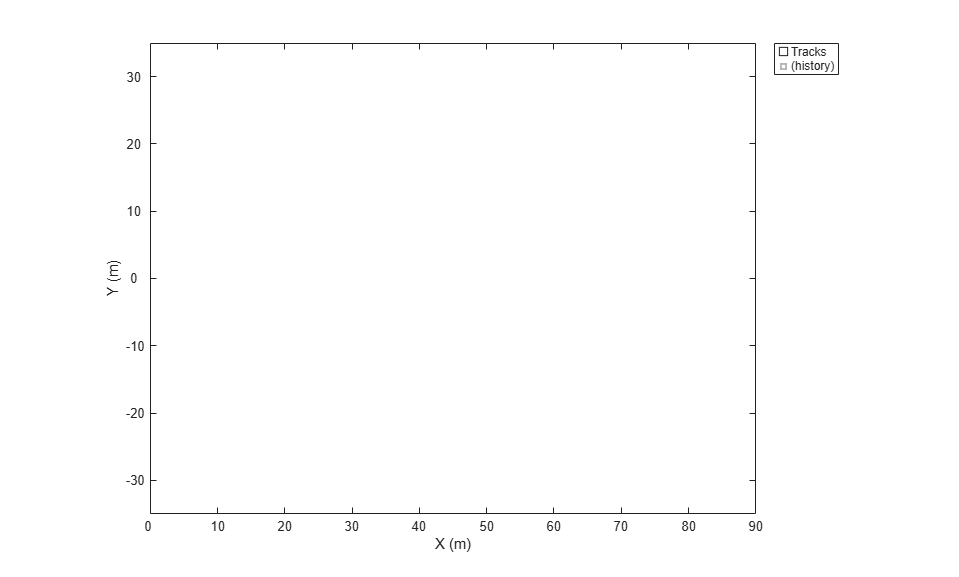Update the track plotter with three tracks labeled `'T1'`, `'T2'`, and `'T3'` with start positions in units of meters all starting at (30, 5, 1) with corresponding velocities (in m/s) of (3, 0, 1), (3, 2, 2) and (3, -3, 5), respectively. Update the tracks with the velocities for ten iterations.

```positions = [30, 5, 1; 30, 5, 1; 30, 5, 1]; velocities = [3, 0, 1; 3, 2, 2; 3, -3, 5]; labels = {'T1','T2','T3'}; for i=1:10 plotTrack(tPlotter, positions, velocities, labels) positions = positions + velocities; end ```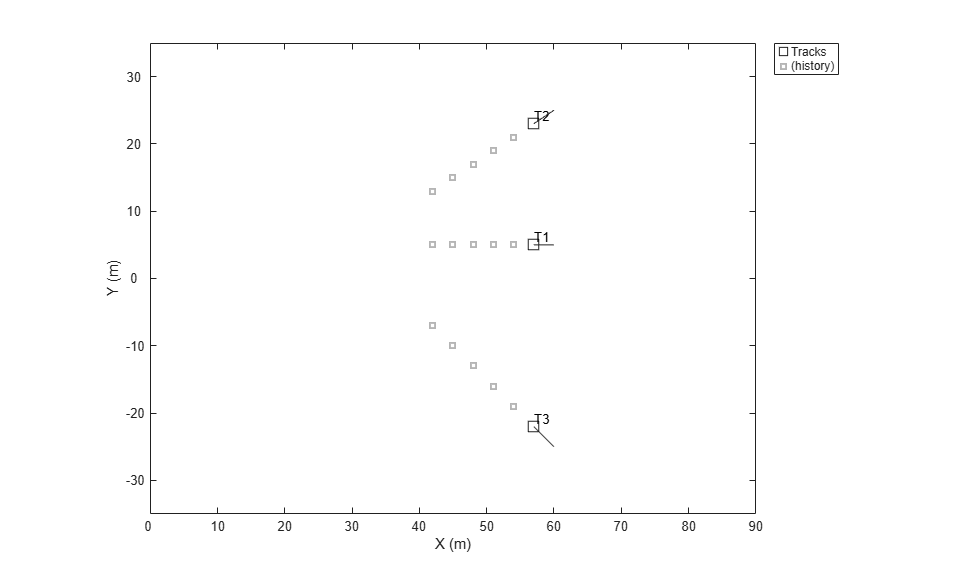This animation loops through all the generated plots.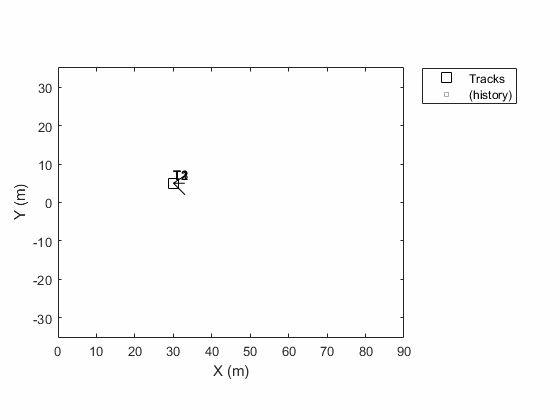Create a theater plot. Create a track plotter with `DisplayName` set to `'Uncertain Track'`.

```tp = theaterPlot('Xlim',[0 5],'Ylim',[0 5]); tPlotter = trackPlotter(tp,'DisplayName','Uncertain Track');```

Update the track plotter with a track at a position in meters (2,2,1) and velocity (in meters/second) of (1,1,3). Also create a random 3-by-3 covariance matrix representing track uncertainties. For purposes of reproducibility, set the random seed to the default value.

``` positions = [2, 2, 1]; velocities = [1, 1, 3]; rng default covariances = randn(3,3);```

Plot the track with the covariances plotted as an ellipsoid.

`plotTrack(tPlotter,positions,velocities,covariances)`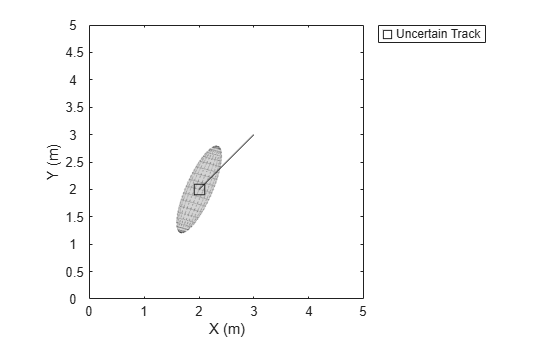## Input Arguments

collapse all

Track plotter, specified as a `trackPlotter` object.

Tracked object positions, specified as an M-by-3 real-valued matrix, where M is the number of objects. Each column of `positions` corresponds to the x-, y-, and z-coordinates of the object locations in meters.

Tracked object velocities, specified as an M-by-3 real-valued matrix, where M is the number of objects. Each column of `velocities` corresponds to the x, y, and z velocities of the objects. If specified, `velocities` must have the same dimensions as `positions`.

Track uncertainties of M tracked objects, specified as a 3-by-3-by-M real-valued array of covariances. The covariances are centered at the track positions, and are plotted as an ellipsoid.

Tracked object labels, specified as a M-by-1 cell array of character vectors, where M is the number of objects. The argument `labels` contains the text labels corresponding to the M objects specified in `positions`. If `labels` is omitted, no labels are plotted.

Unique track identifiers for the M tracked objects, specified as an M-by-1 integer vector, an M-by-1 array of strings, or an M-by-1 cell array of character vectors. The elements of `trackIDs` must be unique.

The `trackIDs` input is ignored when the property ConnectHistory of `tPlotter` is `'off'`. If `trackIDs` is omitted when `'ConnectHistory'` is `'on'`, then the track identifiers are derived from the labels input instead.

Platform dimensions, specified as an M-by-1 array of dimension structures, where M is the number of platforms. The fields of each dimension structure are:

Fields of `Dimensions`

FieldsDescription
`Length`Dimension of a cuboid along the x direction
`Width`Dimension of a cuboid along the y direction
`Height`Dimension of a cuboid along the z direction
`OriginOffset`Position of the platform coordinate frame origin with respect to the cuboid center, specified as a vector of three elements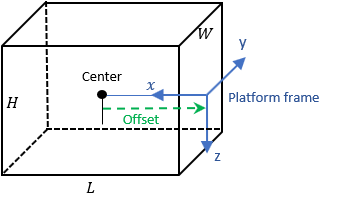Platform orientations, specified as a 3-by-3-by-M array of rotation matrices, or an M-element array of `quaternion` objects.

## Version History

Introduced in R2021a